## NCERT Solutions for Class 10 Maths Ch 8 Introduction to Trigonometry

Chapter 8 Introduction to Trigonometry Class 10 Maths NCERT Solutions will be helpful in completing homework in no time. These NCERT Solutions for Class 10 Maths will be useful in increasing concentration among students. These answers are detailed and prepared according to the latest marking scheme release by CBSE 2020-21.These NCERT Solutions will enhance knowledge of students and they can be able to solve difficult problems in less time.Exercise 8.1

Page No: 181

1. In Δ ABC, right-angled at B, AB = 24 cm, BC = 7 cm. Determine :
(i) sin A, cos A
(ii) sin C, cos C

In Δ ABC,∠B = 90º
By Applying Pythagoras theorem , we get
AC2 = AB2 + BC= (24)2 + 72 = (576+49) cm2 = 625 cm2
⇒ AC = 25
(i) sin A = BC/AC = 7/25    cos A = AB/AC = 24/25
(ii) sin C = AB/AC = 24/25
cos C = BC/AC = 7/25

2.  In Fig. 8.13, find tan P – cot R.

By Applying Pythagoras theorem in ΔPQR , we get
PR2 = PQ2 + QR2 = (13)2 = (12)2 + QR= 169 = 144  + QR2
⇒  QR2 = 25 ⇒  QR = 5 cm
Now,
tan P = QR/PQ = 5/12
cot R = QR/PQ = 5/12
A/q
tan P – cot R = 5/12 - 5/12 = 0

3. If sin A =3/4, calculate cos A and tan A.

Let ΔABC be a right-angled triangle, right-angled at B.
We know that sin A = BC/AC = 3/4
Let BC be 3k and AC will be 4k where k is a positive real number.
By Pythagoras theorem we get,
AC2 = AB2 + BC
(4k)2 = AB2 + (3k)2
16k2 - 9k2 = AB2
AB= 7k2
AB = √7 k
cos A = AB/AC = √7 k/4k = √7/4
tan A = BC/AB = 3k/7 k = 3/7

4. Given 15 cot A = 8, find sin A and sec A.

Let ΔABC be a right-angled triangle, right-angled at B.
We know that cot A = AB/BC = 8/15   (Given)
Let AB be 8k and BC will be 15k where k is a positive real number.
By Pythagoras theorem we get,
AC2 = AB2 + BC
AC2 = (8k)2 + (15k)2
AC2 = 64k2 + 225k2
AC2 = 289k2
AC = 17 k
sin A = BC/AC = 15k/17k = 15/17
sec A = AC/AB = 17k/8 k = 17/8

5. Given sec θ = 13/12, calculate all other trigonometric ratios.

Let ΔABC be a right-angled triangle, right-angled at B.
We know that sec θ = OP/OM = 13/12   (Given)
Let OP be 13k and OM will be 12k where k is a positive real number.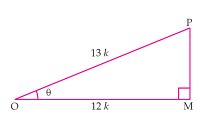By Pythagoras theorem we get,
OP2 = OM2 + MP
(13k)2 = (12k)+ MP
169k2 - 144k2 = MP2
MP2 = 25k2
MP = 5
Now,
sin θ = MP/OP = 5k/13k = 5/13
cos θ = OM/OP = 12k/13k = 12/13
tan θ = MP/OM = 5k/12k = 5/12
cot θ = OM/MP = 12k/5k = 12/5
cosec θ = OP/MP = 13k/5k = 13/5

6.  If ∠A and ∠B are acute angles such that cos A = cos B, then show that ∠A = ∠B.

A/q,
cos A = cos B
Let AD/BD = AC/BC = k
⇒ AD = kBD  .... (i)
⇒ AC = kBC  .... (ii)
By applying Pythagoras theorem in ΔCAD and ΔCBD we get,
CD2 = AC2 - AD2 …. (iii)
and also CD2 = BC2 - BD2 …. (iv)
From equations (iii) and (iv) we get,
AC2 - AD2 = BC2 - BD2
⇒ (kBC)2 - (k BD)2 = BC2 - BD2
⇒ k2 (BC2 - BD2) = BC2 - BD2
⇒ k2 = 1
⇒ k = 1
Putting this value in equation (ii), we obtain
AC = BC
⇒ ∠A = ∠B  (Angles opposite to equal sides of a triangle are equal-isosceles triangle)

7. If cot θ =7/8, evaluate :
(i)(1+sin θ )(1-sin θ)/(1+cos θ)(1-cos θ)
(ii) cot2θ

A/q,
cot θ = BC/AB = 7/8
Let BC = 7k and AB = 8k, where k is a positive real number.
By Pythagoras theorem in ΔABC we get.
AC2 = AB2 + BC
AC2 = (8k)2 + (7k)2
AC2 = 64k2 + 49k2
AC2 = 113k2
AC = √113 k
sin θ = AB/AC = 8k/113 k = 8/113
and cos θ = BC/AC = 7k/113 k = 7/113
(i) (1+sin θ )(1-sin θ)/(1+cos θ)(1-cos θ) = (1-sin2θ)/(1-cos2θ) = {1 - (8/113)2}/{1 - (7/113)2}
= {1 - (64/113)}/{1 - (49/113)} = {(113 - 64)/113}/{(113 - 49)/113} = 49/64
(ii) cot2θ = (7/8)2 = 49/64

8.  If 3cot A = 4/3 , check whether (1-tan2A)/(1+tan2A) = cos2A – sin2A or not.Let ΔABC in which ∠B = 90º,
A/q,
cot A = AB/BC = 4/3
Let AB = 4k and BC = 3k, where k is a positive real number.
By Pythagoras theorem in ΔABC we get.
AC2 = AB2 + BC
AC2 = (4k)2 + (3k)2
AC2 = 16k2 + 9k2
AC2 = 25k2
AC = 5k
tan A = BC/AB = 3/4
sin A = BC/AC = 3/5
cos A = AB/AC = 4/5
L.H.S. = (1-tan2A)/(1+tan2A) = 1- (3/4)2/1+ (3/4)= (1- 9/16)/(1+ 9/16) = (16-9)/(16+9) = 7/25
R.H.S. = cos2A – sin2A = (4/5)2 - (3/4)2 = (16/25) - (9/25) = 7/25
R.H.S. = L.H.S.
Hence,  (1-tan2A)/(1+tan2A) = cos2A – sin2A

9. In triangle ABC, right-angled at B, if tan A =1/3 find the value of:
(i) sin A cos C + cos A sin C
(ii) cos A cos C – sin A sin C

Let ΔABC in which ∠B = 90º,
A/q,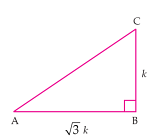tan A = BC/AB = 1/3
Let AB = √3 k and BC = k, where k is a positive real number.
By Pythagoras theorem in ΔABC we get.
AC2 = AB2 + BC
AC2 = (√3 k)2 + (k)2
AC2 = 3k2 + k2
AC2 = 4k2
AC = 2k
sin A = BC/AC = 1/2                   cos A = AB/AC = √3/2 ,
sin C = AB/AC = √3/2                   cos A = BC/AC = 1/2
(i) sin A cos C + cos A sin C = (1/2×1/2) + (√3/2×√3/2) = 1/4+3/4 = 4/4 = 1
(ii) cos A cos C – sin A sin C = (√3/2×1/2) - (1/2×√3/2) = √3/4 - √3/4 = 0

10. In Δ PQR, right-angled at Q, PR + QR = 25 cm and PQ = 5 cm. Determine the values of sin P, cos P and tan P.

Given that, PR + QR = 25 , PQ = 5
Let PR be x.  ∴ QR = 25 - x
By Pythagoras theorem ,
PR2 = PQ2 + QR2
x2 = (5)2 + (25 - x)2
x2 = 25 + 625 + x2 - 50x
50x = 650
x = 13
∴ PR = 13 cm
QR = (25 - 13) cm = 12 cm
sin P = QR/PR = 12/13
cos P = PQ/PR = 5/13
tan P = QR/PQ = 12/5

11.  State whether the following are true or false. Justify your answer.
(i) The value of tan A is always less than 1.
(ii) sec A = 12/5 for some value of angle A.
(iii) cos A is the abbreviation used for the cosecant of angle A.
(iv) cot A is the product of cot and A.
(v) sin θ = 4/3 for some angle θ.

In ΔABC in which ∠B = 90º,
AB = 3, BC = 4 and AC = 5
Value of tan A = 4/3 which is greater than.
The triangle can be formed with sides equal to 3, 4 and hypotenuse = 5 as
it will follow the Pythagoras theorem.AC2 = AB2 + BC
52 = 32 + 42
25 = 9 + 16
25 = 25

(ii) True.
Let a ΔABC in which ∠B = 90º,AC be 12k and AB be 5k, where k is a positive real number.
By Pythagoras theorem we get,
AC2 = AB2 + BC
(12k)2 = (5k)2 + BC
BC+ 25k= 144k2
BC= 119k2
Such a triangle is possible as it will follow the Pythagoras theorem.

(iii) False.
Abbreviation used for cosecant of angle A is cosec A.cos A is the abbreviation used for cosine of angle A.

(iv) False.
cot A is not the product of cot and A. It is the cotangent of ∠A.

(v) False.
sin θ = Height/Hypotenuse
We know that in a right angled triangle, Hypotenuse is the longest side.
∴ sin θ will always less than 1 and it can never be 4/3 for any value of θ.

Excercise 8.2

Page No: 187

1. Evaluate the following :
(i) sin 60° cos 30° + sin 30° cos 60° (ii) 2 tan245° + cos230° – sin260°
(iii) cos 45°/(sec 30° + cosec 30°)    (iv) (sin 30° + tan 45° – cosec 60°)/(sec 30° + cos 60° + cot 45°)
(v) (5cos260° + 4sec230° - tan245°)/(sin230° + cos230°)

(i) sin 60° cos 30° + sin 30° cos 60°
=  (√3/2×√3/2) + (1/2×1/2) = 3/4 + 1/4 = 4/4 = 1

(ii) 2 tan245° + cos230° – sin260°
= 2×(1)+ (√3/2)2 - (√3/2)= 2

(iii) cos 45°/(sec 30° + cosec 30°)
= 1/√2/(2/√3 + 2) = 1/√2/{(2+2√3)/3)
= √3/2×(2+2√3) = √3/(2√2+2√6)
= √3(2√6-2√2)/(2√6+2√2)(2√6-2√2)
= 2√3(√6-√2)/(2√6)2-(2√2)2
=  2√3(√6-√2)/(24-8) =  2√3(√6-√2)/16
= √3(√6-√2)/8 = (√18-√6)/8 = (3√2-√6)/8

(iv) (sin 30° + tan 45° – cosec 60°)/(sec 30° + cos 60° + cot 45°)
= (1/2+1-2/√3)/(2/√3+1/2+1)
= (3/2-2/√3)/(3/2+2/√3)
= (3√3-4/2√3)/(3√3+4/2√3)
= (3√3-4)/(3√3+4)
= (3√3-4)(3√3-4)/(3√3+4)(3√3-4)
= (3√3-4)2/(3√3)2-(4)2
= (27+16-24√3)/(27-16)
= (43-24√3)/11]

(v) (5cos260° + 4sec230° - tan245°)/(sin230° + cos230°)
= 5(1/2)2+4(2/√3)2-12/(1/2)2+(√3/2)2
= (5/4+16/3-1)/(1/4+3/4)
= (15+64-12)/12/(4/4)
= 67/12

2. Choose the correct option and justify your choice :
(i) 2tan 30°/1+tan230° =
(A) sin 60°            (B) cos 60°          (C) tan 60°            (D) sin 30°
(ii) 1-tan245°/1+tan245° =
(A) tan 90°            (B) 1                    (C) sin 45°            (D) 0
(iii)  sin 2A = 2 sin A is true when A =
(A) 0°                   (B) 30°                  (C) 45°                 (D) 60°
(iv) 2tan30°/1-tan230° =
(A) cos 60°          (B) sin 60°             (C) tan 60°           (D) sin 30°

(i) (A) is correct.
2tan 30°/1+tan230° = 2(1/√3)/1+(1/√3)2
= (2/√3)/(1+1/3) = (2/√3)/(4/3)
= 6/4√3 = √3/2 = sin 60°

(ii)  (D) is correct.
1-tan245°/1+tan245° = (1-12)/(1+12)
= 0/2 = 0

(iii) (A) is correct.
sin 2A = 2 sin A is true when A =
= As sin 2A = sin 0° = 0
2 sin A = 2sin 0° = 2×0 = 0
or,
sin 2A = 2sin A cos A
⇒2sin A cos A = 2 sin A
⇒ 2cos A = 2 ⇒ cos A = 1
⇒ A = 0°

(iv) (C) is correct.
2tan30°/1-tan230° =  2(1/√3)/1-(1/√3)2
= (2/√3)/(1-1/3) = (2/√3)/(2/3) = √3 = tan 60°

3. If tan (A + B) = √3 and tan (A – B) = 1/3; 0° < A + B ≤ 90°; A > B, find A and B.

tan (A + B) = √3
⇒ tan (A + B) = tan 60°
⇒ (A + B) = 60° ... (i)
tan (A – B) = 1/3
⇒ tan (A - B) = tan 30°
⇒ (A - B) = 30° ... (ii)
Adding (i) and (ii), we get
A + B + A - B = 60° + 30°
2A = 90°
A= 45°
Putting the value of A in equation (i)
45° + B = 60°
⇒ B = 60° - 45°
⇒ B = 15°
Thus, A = 45° and B = 15°

4. State whether the following are true or false. Justify your answer.
(i) sin (A + B) = sin A + sin B.
(ii) The value of sin θ increases as θ increases.
(iii) The value of cos θ increases as θ increases.
(iv) sin θ = cos θ for all values of θ.
(v) cot A is not defined for A = 0°.

(i) False.
Let A = 30° and B = 60°, then
sin (A + B) = sin (30° + 60°) = sin 90° = 1 and,
sin A + sin B = sin 30° + sin 60°
= 1/2 + √3/2 = 1+√3/2

(ii) True.
sin 0° = 0
sin 30° = 1/2
sin 45° = 1/2
sin 60° = √3/2
sin  90° = 1
Thus the value of sin θ increases as θ increases.

(iii) False.
cos 0° = 1
cos 30° = √3/2
cos 45° = 1/2
cos 60° = 1/2
cos 90° = 0
Thus the value of cos θ decreases as θ increases.

(iv) True.
cot A = cos A/sin A
cot 0° = cos 0°/sin 0° = 1/0 = undefined.

Excercise 8.3

Page No : 189

1. Evaluate :
(i) sin 18°/cos 72°        (ii) tan 26°/cot 64°        (iii)  cos 48° – sin 42°       (iv)  cosec 31° – sec 59°

(i) sin 18°/cos 72°
= sin (90° - 18°) /cos 72°
= cos 72° /cos 72° = 1

(ii) tan 26°/cot 64°
= tan (90° - 36°)/cot 64°
= cot 64°/cot 64° = 1

(iii) cos 48° - sin 42°
= cos (90° - 42°) - sin 42°
= sin 42° - sin 42° = 0

(iv) cosec 31° - sec 59°
= cosec (90° - 59°) - sec 59°
= sec 59° - sec 59° = 0

2.  Show that :
(i) tan 48° tan 23° tan 42° tan 67° = 1
(ii) cos 38° cos 52° – sin 38° sin 52° = 0

(i) tan 48° tan 23° tan 42° tan 67°
= tan (90° - 42°) tan (90° - 67°) tan 42° tan 67°
= cot 42° cot 67° tan 42° tan 67°
= (cot 42° tan 42°) (cot 67° tan 67°) = 1×1 = 1

(ii) cos 38° cos 52° - sin 38° sin 52°
= cos (90° - 52°) cos (90°-38°) - sin 38° sin 52°
= sin 52° sin 38° - sin 38° sin 52° = 0

3. If tan 2A = cot (A – 18°), where 2A is an acute angle, find the value of A.

A/q,
tan 2A = cot (A- 18°)
⇒ cot (90° - 2A) = cot (A -18°)
Equating angles,
⇒ 90° - 2A = A- 18° ⇒ 108° = 3A
⇒ A = 36°

4.  If tan A = cot B, prove that A + B = 90°.

A/q,
tan A = cot B
⇒ tan A = tan (90° - B)
⇒ A = 90° - B
⇒ A + B = 90°

5. If sec 4A = cosec (A – 20°), where 4A is an acute angle, find the value of A.

A/q,
sec 4A = cosec (A - 20°)
⇒ cosec (90° - 4A) = cosec (A - 20°)
Equating angles,
90° - 4A= A- 20°
⇒ 110° = 5A
⇒ A = 22°

Page No : 190

6. If A, B and C are interior angles of a triangle ABC, then show that
sin (B+C/2) = cos A/2

In a triangle, sum of all the interior angles
A + B + C = 180°
⇒ B + C = 180° - A
⇒ (B+C)/2 = (180°-A)/2
⇒ (B+C)/2 = (90°-A/2)
⇒ sin (B+C)/2 = sin (90°-A/2)
⇒ sin (B+C)/2 = cos A/2

7. Express sin 67° + cos 75° in terms of trigonometric ratios of angles between 0° and 45°.

sin 67° + cos 75°
= sin (90° - 23°) + cos (90° - 15°)
= cos 23° + sin 15°

Excercise 8.4

Page No : 193

1. Express the trigonometric ratios sin A, sec A and tan A in terms of cot A.

cosec2A - cot2A = 1
⇒ cosec2A = 1 + cot2A
⇒ 1/sin2A = 1 + cot2A
⇒sin2A = 1/(1+cot2A)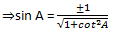Now,
sin2A = 1/(1+cot2A)
⇒ 1 - cos2A = 1/(1+cot2A)
⇒cos2A = 1 - 1/(1+cot2A)
⇒cos2A = (1-1+cot2A)/(1+cot2A)
⇒ 1/sec2A = cot2A/(1+cot2A)
⇒ secA = (1+cot2A)/cot2Aalso,
tan A = sin A/cos A and cot A = cos A/sin A
⇒ tan A = 1/cot A

2. Write all the other trigonometric ratios of ∠A in terms of sec A.

We know that,
sec A = 1/cos A
⇒ cos A = 1/sec A
also,
cos2A + sin2A = 1
⇒  sin2A = 1 - cos2A
⇒  sin2A = 1 - (1/sec2A)
⇒  sin2A = (sec2A-1)/sec2A

also,
sin A = 1/cosec A
⇒cosec A = 1/sin A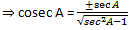Now,
sec2A - tan2A = 1
⇒ tan2A = sec2A + 1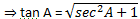also,
tan A = 1/cot A
⇒ cot A = 1/tan A3. Evaluate :
(i) (sin263° + sin227°)/(cos217° + cos273°)
(ii)  sin 25° cos 65° + cos 25° sin 65°

(i) (sin263° + sin227°)/(cos217° + cos273°)
= [sin2(90°-27°) + sin227°]/[cos2(90°-73°) + cos273°)]
= (cos227° + sin227°)/(sin227° + cos273°)
= 1/1 =1                       (∵ sin2A + cos2A = 1)

(ii) sin 25° cos 65° + cos 25° sin 65°
= sin(90°-25°) cos 65° + cos(90°-65°) sin 65°
= cos 65° cos 65° + sin 65° sin 65°
= cos265° + sin265° = 1

4. Choose the correct option. Justify your choice.
(i) 9 sec2A - 9 tan2A =
(A) 1                 (B) 9              (C) 8                (D) 0
(ii) (1 + tan θ + sec θ) (1 + cot θ - cosec θ)
(A) 0                 (B) 1              (C) 2                (D) - 1
(iii) (secA + tanA) (1 - sinA) =
(A) secA           (B) sinA        (C) cosecA      (D) cosA
(iv) 1+tan2A/1+cot2A =
(A) sec2A                 (B) -1              (C) cot2A                (D) tan2A

(i) (B) is correct.
9 sec2A - 9 tan2A
= 9 (sec2A - tan2A)
= 9×1 = 9             (∵ sec2 A - tan2 A = 1)

(ii) (C) is correct
(1 + tan θ + sec θ) (1 + cot θ - cosec θ)
= (1 + sin θ/cos θ + 1/cos θ) (1 + cos θ/sin θ - 1/sin θ)
= (cos θ+sin θ+1)/cos θ × (sin θ+cos θ-1)/sin θ
= (cos θ+sin θ)2-12/(cos θ sin θ)
= (cos2θ + sin2θ + 2cos θ sin θ -1)/(cos θ sin θ)
= (1+ 2cos θ sin θ -1)/(cos θ sin θ)
= (2cos θ sin θ)/(cos θ sin θ) = 2

(iii) (D) is correct.
(secA + tanA) (1 - sinA)
= (1/cos A + sin A/cos A) (1 - sinA)
= (1+sin A/cos A) (1 - sinA)
= (1 - sin2A)/cos A
= cos2A/cos A = cos A

(iv) (D) is correct.
1+tan2A/1+cot2
= (1+1/cot2A)/1+cot2A
= (cot2A+1/cot2A)×(1/1+cot2A)
= 1/cot2A = tan2A

5. Prove the following identities, where the angles involved are acute angles for which the
expressions are defined.
(i) (cosec θ - cot θ)= (1-cos θ)/(1+cos θ)
(ii) cos A/(1+sin A) + (1+sin A)/cos A = 2 sec A
(iii) tan θ/(1-cot θ) + cot θ/(1-tan θ) = 1 + sec θ cosec θ
[Hint : Write the expression in terms of sin θ and cos θ]
(iv) (1 + sec A)/sec A = sin2A/(1-cos A)
[Hint : Simplify LHS and RHS separately]
(v) (cos A–sin A+1)/(cos A+sin A–1) = cosec A + cot A,using the identity cosec2A = 1+cot2A.

(vii) (sin θ - 2sin3θ)/(2cos3θ-cos θ) = tan θ
(viii) (sin A + cosec A)+ (cos A + sec A)2 = 7+tan2A+cot2A
(ix) (cosec A – sin A)(sec A – cos A) = 1/(tan A+cotA)
[Hint : Simplify LHS and RHS separately]
(x) (1+tan2A/1+cot2A) = (1-tan A/1-cot A)2 = tan2A

(i) (cosec θ - cot θ)= (1-cos θ)/(1+cos θ)
L.H.S. =  (cosec θ - cot θ)2
= (cosec2θ + cot2θ - 2cosec θ cot θ)
= (1/sin2θ + cos2θ/sin2θ - 2cos θ/sin2θ)
= (1 + cos2θ - 2cos θ)/(1 - cos2θ)
= (1-cos θ)2/(1 - cosθ)(1+cos θ)
= (1-cos θ)/(1+cos θ) = R.H.S.

(ii)  cos A/(1+sin A) + (1+sin A)/cos A = 2 sec A
L.H.S. = cos A/(1+sin A) + (1+sin A)/cos A
= [cos2A + (1+sin A)2]/(1+sin A)cos A
= (cos2A + sin2A + 1 + 2sin A)/(1+sin A)cos A
= (1 + 1 + 2sin A)/(1+sin A)cos A
= (2+ 2sin A)/(1+sin A)cos A
= 2(1+sin A)/(1+sin A)cos A
= 2/cos A = 2 sec A = R.H.S.

(iii) tan θ/(1-cot θ) + cot θ/(1-tan θ) = 1 + sec θ cosec θ
L.H.S. = tan θ/(1-cot θ) + cot θ/(1-tan θ)
= [(sin θ/cos θ)/1-(cos θ/sin θ)] + [(cos θ/sin θ)/1-(sin θ/cos θ)]
= [(sin θ/cos θ)/(sin θ-cos θ)/sin θ] + [(cos θ/sin θ)/(cos θ-sin θ)/cos θ]
= sin2θ/[cos θ(sin θ-cos θ)] + cos2θ/[sin θ(cos θ-sin θ)]
= sin2θ/[cos θ(sin θ-cos θ)] - cos2θ/[sin θ(sin θ-cos θ)]
= 1/(sin θ-cos θ) [(sin2θ/cos θ) - (cos2θ/sin θ)]
= 1/(sin θ-cos θ) × [(sin3θ - cos3θ)/sin θ cos θ]
= [(sin θ-cos θ)(sin2θ+cos2θ+sin θ cos θ)]/[(sin θ-cos θ)sin θ cos θ]
= (1 + sin θ cos θ)/sin θ cos θ
= 1/sin θ cos θ + 1
= 1 + sec θ cosec θ = R.H.S.

(iv)  (1 + sec A)/sec A = sin2A/(1-cos A)
L.H.S. = (1 + sec A)/sec A
= (1 + 1/cos A)/1/cos A
= (cos A + 1)/cos A/1/cos A
= cos A + 1
R.H.S. = sin2A/(1-cos A)
= (1 - cos2A)/(1-cos A)
= (1-cos A)(1+cos A)/(1-cos A)
= cos A + 1
L.H.S. = R.H.S.

(v) (cos A–sin A+1)/(cos A+sin A–1) = cosec A + cot A,using the identity cosec2A = 1+cot2A.
L.H.S. = (cos A–sin A+1)/(cos A+sin A–1)
Dividing Numerator and Denominator by sin A,
= (cos A–sin A+1)/sin A/(cos A+sin A–1)/sin A
= (cot A - 1 + cosec A)/(cot A+ 1 – cosec A)
= (cot A - cosec2A + cot2A + cosec A)/(cot A+ 1 – cosec A) (using cosec2A - cot2A = 1)
= [(cot A + cosec A) - (cosec2A - cot2A)]/(cot A+ 1 – cosec A)
= [(cot A + cosec A) - (cosec A + cot A)(cosec A - cot A)]/(1 – cosec A + cot A)
=  (cot A + cosec A)(1 – cosec A + cot A)/(1 – cosec A + cot A)
=  cot A + cosec A = R.H.S.Dividing Numerator and Denominator of L.H.S. by cos A,= sec A + tan A = R.H.S.

(vii) (sin θ - 2sin3θ)/(2cos3θ-cos θ) = tan θ
L.H.S. = (sin θ - 2sin3θ)/(2cos3θ - cos θ)
= [sin θ(1 - 2sin2θ)]/[cos θ(2cos2θ- 1)]
= sin θ[1 - 2(1-cos2θ)]/[cos θ(2cos2θ -1)]
= [sin θ(2cos2θ -1)]/[cos θ(2cos2θ -1)]
= tan θ = R.H.S.

(viii) (sin A + cosec A)+ (cos A + sec A)2 = 7+tan2A+cot2A
L.H.S. = (sin A + cosec A)+ (cos A + sec A)2
= (sin2A + cosec2A + 2 sin A cosec A) + (cos2A + sec2A + 2 cos A sec A)
= (sin2A + cos2A) + 2 sin A(1/sin A) + 2 cos A(1/cos A) + 1 + tan2A + 1 + cot2A
= 1 + 2 + 2 + 2 + tan2A + cot2A
= 7+tan2A+cot2A = R.H.S.

(ix) (cosec A – sin A)(sec A – cos A) = 1/(tan A+cotA)
L.H.S. = (cosec A – sin A)(sec A – cos A)
= (1/sin A - sin A)(1/cos A - cos A)
= [(1-sin2A)/sin A][(1-cos2A)/cos A]
= (cos2A/sin A)×(sin2A/cos A)
= cos A sin A
R.H.S. = 1/(tan A+cotA)
= 1/(sin A/cos A +cos A/sin A)
= 1/[(sin2A+cos2A)/sin A cos A]
= cos A sin A
L.H.S. = R.H.S.

(x)  (1+tan2A/1+cot2A) = (1-tan A/1-cot A)2 = tan2A
L.H.S. = (1+tan2A/1+cot2A)
= (1+tan2A/1+1/tan2A)
= 1+tan2A/[(1+tan2A)/tan2A]
= tan2A

Go Back To NCERT Solutions for Class 10 Maths

### NCERT Solutions for Class 10 Maths Chapter 8 Introduction to Trigonometry

Chapter 8 Class 10 Maths NCERT Solutions will help students in preparing well before the examinations and guide students in a better way. There are total 5 topics in the chapter which will enhance your knowledge regarding trigonometric identities.

• Introduction: Trigonometry is the study of relationships between the sides and angles of a triangle. In today's world,  most of the technologically advanced methods used in Engineering and Physical Sciences are based on trigonometrical concepts.

• Trigonometric Ratios: We will study some ratios of the sides of a right triangle with respect to its acute angles, called trigonometric ratios of the angle. We will limit our study to acute angles only.

• Trigonometric Ratios of Some Specific Angles: We will be finding the values of the trigonometric ratios for 30°, 45°, 60° and also 0°.

• Trigonometric Ratios of Complementary Angles: Two angles are said to be complementary
if their sum equals 90°. You need to find the vale of specific angle through the situation given.

• Trigonometric Identities: An equation involving trigonometric ratios of an angle is called a trigonometric identity, if it is true for all values of the angle(s) involved.

There are total 4 exercises in the NCERT Solutions for Class 10 Maths Chapter 8 Introduction to Trigonometry which are great source of inculcating correct learning habits among students. You can also find exercisewise NCERT Solutions for Chapter 8 Class 10 Maths by clicking on the links given below.

NCERT Solutions for Class 10 Maths Chapters:

 Chapter 1 Real Numbers Chapter 2 Polynomials Chapter 3 Pair of Linear Equations in Two Variables Chapter 4 Quadratic Equations Chapter 5 Arithmetic Progressions Chapter 6 Triangles Chapter 7 Coordinate Geometry Chapter 9 Some Applications Of Trigonometry Chapter 10 Circles Chapter 11 Constructions Chapter 12 Areas Related To Circles Chapter 13 Surface Areas And Volumes Chapter 14 Statistics Chapter 15 Probability

FAQ on Chapter 8 Introduction to Trigonometry

#### How many exercises in Chapter 8 Introduction to Trigonometry

There are only 4 exercises in the Chapter 8 Class 10 Maths. The answers provided here are accurate and will provide opportunities to learn new things.

#### If tan A = cot B, prove that A + B = 90°.

∵ tan A = cot B
∴ tan A = tan (90° − B)
⇒ A = 90° − B
⇒ A + B = 90°.

#### What is the value of sinθ. cos(90° − θ) + cosθ . sin(90° − θ)?

sinθ · cos(90° − θ) + cosθ · sin(90° − θ) = sinθ · sinθ + cosθ · cosθ
[∵ cos(90° − θ) = sinθ , sin(90° − θ) = cos θ]
= sin2 θ + cos2 θ
= 1

#### Express sin 67° + cos 75° in terms of ratios of angles between 0° and 45°.

∵ 67° = 90° − 23° and 75° = 90° − 15°

∴ sin 67° + cos 75° = sin (90° − 23°) + cos (90° − 15°)  = cos 23° + sin 15°.